## TOP 100

The following General Curriculum Multi-Subject MTEL (03,)  Challenge #1 ~ The TOP 100 ~  is designed to help you review the TOP 100 Terms in preparation for the 2-DAY EARLY Multi-Subject (03) Workshop.  See video directions above for pre-workshop home-study usage.

SCIENCE

2

Scientific Inquiry & Engineering

Human Body 101

Life Science and Biology 101

Engineering and Technology

Earth & Planets  101

###### 3

Ancient Civilizations

Medieval Age

European Exploration

Age of Enlightenment

Geography

###### 4

Colonies &  American Revolution

U.S. Government & Economy

Western Expansion

Pre & Post Civil War

The Gilded Age

20th Century

###### Rise of Totalitarianism 1920-45

Cold War & US Policies 1946-80s

The Civil Rights Movement

The 80's

Physics 101

Chemistry 101

Constants and Formulas in Chemistry

## EXAMPLE 2: Pearl Harbor 1941: Japan bomb's Pearl Harbor,   USA enters WW2.## Boyle's Law

### Boyle’s Law describes the inverse proportional relationship between pressure and volume at a constant temperature and a fixed amount of gas. This law came from a manipulation of the Ideal Gas Law.## Charles's Law

### Charles's Law describes the directly proportional relationship between the volume and temperature (in Kelvin) of a fixed amount of gas, when the pressure is held constant.### Volume of a gas is directly proportional to the amount of gas at a constant temperature and pressure.## Ideal Gas Law

### The ideal gas law, also called the general gas equation, is the equation of state of a hypothetical ideal gas. It is a good approximation of the behavior of many gases under many conditions, although it has several limitations.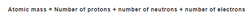## Atomic Mass

### Atomic mass is the total of the masses of the electrons, neutrons, and protons in an atom, or in a group of atoms, the average mass.## Dalton's Law Formula

### Dalton’s Law of partial pressure states that “the total pressure exerted by the mixture of gases is the sum of all the partial pressure of the individual gases.”## Bond Order Formula

### The bond order shows the number of chemical bonds present between a pair of atoms.## Boiling Point Formula

### The boiling point is the temperature at which a liquid starts to boil and transforms to vapor.## Grams to Moles Formula

### The common or usual weight measurements in chemistry is figured out using moles.## pH Formula

### pH is a measure of the amount of activity of hydrogen ions (H+) in a solution and thus its alkalinity or acidity.## Molar Volume

### The Molar Volume, represented by Vm, is the volume occupied by one mole of a substance which can be a chemical element or a chemical compound at Standard Temperature and Pressure (STP).## Number of Moles Formula

### A mole of a defined substance is defined as the mass of that substance containing the same number of fundamental units just like atoms in 12.0 g of 12C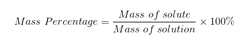## Mass Percent Formula

### The Mass percent is a way of expressing a concentration or describing the component in a particular mixture.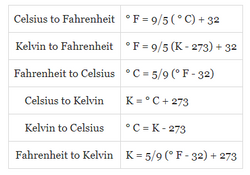## Temperature Conversion

### The three common temperature scales are Celsius, Fahrenheit, and Kelvin. Each scale has its uses, so it's likely you'll encounter them and need to convert between them.## Percent Error Formula

### When you calculate results that are aiming for known values, the percent error formula is useful tool for determining the precision of your calculations.## Force

### Force is the mass of an object, multiplied by its acceleration## Weight

### Weight is a force that acts at all times on all objects near Earth.## Density

### Density is a measure of relative compactness, or how heavy an object is relative to its size## Acceleration

### Acceleration is a measure of how quickly the velocity of an object changes## Velocity

### Velocity is a measure of how quickly an object moves.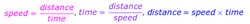## Distance, Speed, and Time

### Speed is a measure of how quickly an object moves from one place to another. It is equal to the distance traveled divided by the time. It is possible to find any of these three values using the other two## Displacement

### Displacement is the change in an object's position from the origin## Kinetic energy

### Kinetic energy is the energy of moving objects. An object's kinetic energy depends on the object's mass and velocity.## Potential energy

### Potential energy is energy that is stored in a system. There is the possibility, or potential, for it to be converted to kinetic energy.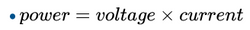## Power

### Power is a rate at which work is done, or energy is used.## Current

### The rate of flow of charge through a cross section of some region of a metallic material is called the electric current.## Work

### Work is the result when a force acts on an object and moves it by some distance## Pressure

### Pressure is a force per unit area that acts on an object.## Momentum

### Momentum is a quantity with a value and a direction## Impulse

### An impulse is a force applied for a specified period of time.## Stress

### Stress is a measure of the internal force an object is experiencing per unit cross-sectional area## Pythagoras Theorem

### The square of the hypotenuse (the side opposite the right angle) is equal to the sum of the squares of the other two sides.## Lever

### A lever is a bar resting on a pivot. Force (effort) applied at one point is transmitted across the pivot (fulcrum) to another point which moves an object (load).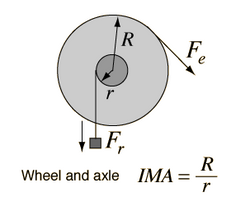## Wheel and Axle

### The wheel and axle is essentially a modified lever, but it can move a load farther than a lever can. The center of the axle serves as a fulcrum.## Pulley

### A pulley is a wheel over which a rope or belt is passed. It is also a form of the wheel and axle.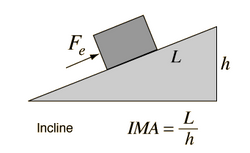## Inclined Plane

### The inclined plane is a simple device that hardly looks like a machine at all. The mechanical advantage increases as the slope of the incline decreases.## Wedge

### The wedge is an adaptation of the inclined plane. It can be used to raise a heavy load over a short distance or to split a log.